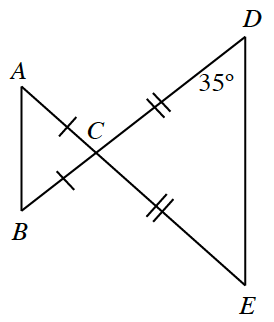### Home > INT2 > Chapter 6 > Lesson 6.2.6 > Problem6-103

6-103.Copy the diagram at right onto your paper.

1. What are the measures of all the angles in the diagram?

The triangles in the diagram are isosceles, therefore their base angles are congruent.

2. Make a flowchart showing that the triangles are similar.

3.Cheri and Roberta notice that their similarity statements for part (b) are not the same. Cheri stated $ΔABC$ ~ $ΔDEC$, while Roberta wrote $ΔABC$ ~ $ΔEDC$. Who is correct? Or are they both correct? Explain your reasoning.

They are both correct. Since both triangles are isosceles, we cannot tell if one is the reflection or the rotation of the other (after dilation).